Next: The B-tube. Up: Alan Turing's Manual for Previous: The multiplier and the

Subsections

# The logical operations

There are a number of operations which can be applied to rows of digits and which have no particular relation to the interpretation of those rows as numbers. The only ones which we use are what we call the logical operations', on account of a connection with the calculus of propositions in logic. In order to bring out this connection one should identify 1 with truth' and 0 with falsity'. The symbol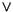' may be read as or',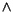' may be read as and',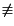' as not equivalent to' [and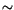as not']. These symbols satisfy the equations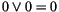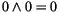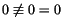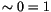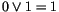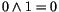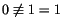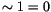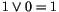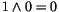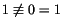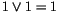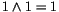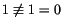However we shall apply these symbols to rows of digits rather than to single digits. The value for each digit is then to be calculated by means of the above table from the corresponding digits of the arguments, e.g.,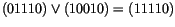The main application of these operations occurs where several different pieces of information are packed into one line. For this purpose the operation' is of the most use. It can be used for breaking a line up into its various significant parts. The operation' may also be used for combining parts together, but this can usually also be done with the aid of addition. The operation' can be obtained by using' in combination with a row of 1's. [The following function codes are used to call for these operations:

 Function symbol Equations TD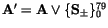TR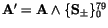TJ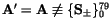TS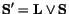,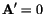TE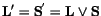,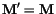where, as before, we define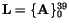and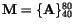. Note that the TS and TE operations are deprecated as disadvantageous'' on p., where the programmer is enjoined against their use.]

## Example of use of `'

The name of a certain line is contained in the first two characters of . It is required to transfer this line to /C.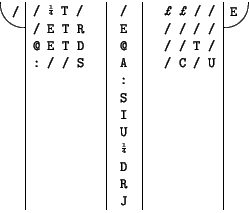[Note once again that ://S at A/ writes a /C/U instruction from AE into S/, in addition to writing a T/ instruction into :/, since it is a long-line store.]